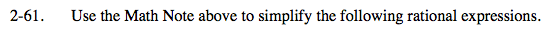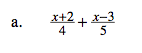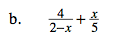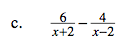### Home > PC > Chapter 2 > Lesson 2.2.2 > Problem2-61

2-61.$\frac{x+2}{4}\cdot\frac{5}{5}+\frac{x-3}{5}\cdot\frac{4}{4}$$\frac{4}{2-x}\cdot\frac{5}{5}+\frac{x}{5}\cdot\frac{2-x}{2-x}$$\frac{6}{x+2}\cdot\frac{x-2}{x-2}-\frac{4}{x-2}\cdot\frac{x+2}{x+2}$

$\frac{6x-12}{x^2-4}-\frac{4x+8}{x^2-4}$